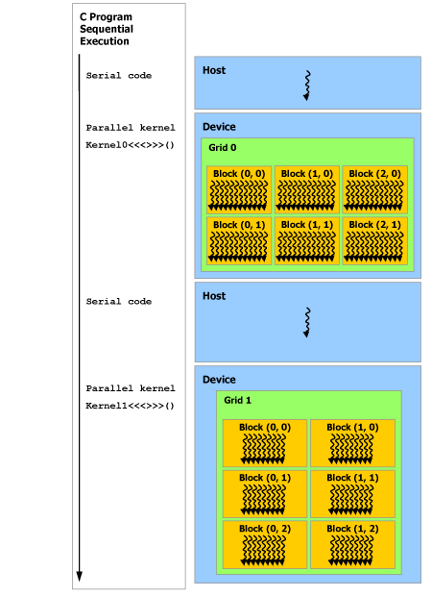# Hello CUDA - CUDA程序简单入门

#include <stdio.h>
int main() {
printf("Hello CUDA!\\n");
return 0;
}

nvcc hello_cuda.cu -o hello_cuda.out

Hello CUDA!

void function_call3() {
}

__device__ void function_call2() {
}

__global__ void function_call1() {
function_call2();               // success
}

int main() {
function_call1<<<1,1>>>();      // success
// function_call2<<<1, 1>>>();  // error: a __device__ function call cannot be configured
function_call3();               // success
return 0;
}

CUDA程序主要有两个部分，CPU上运行的程序和GPU上的程序。这些特殊的关键字就是为了说明后面定义的函数（这些关键字也可以修饰变量）在何处运行。main函数作为入口函数，之前没有任何的修饰，是很正常的CPU程序。同理function_call3也是CPU的程序(CPU上的程序也可以用__host__来修饰)。function_call1__global__修饰，意思是该程序可以被任何程序所调用（CPU或GPU），但是是在GPU上运行。function_call2__device__修饰，表示该程序是GPU上的程序并且只能被GPU程序所调用。理解调用规则了吗？<<<1, 1>>>这个符号，这也是对C/C++的拓展，这两个数字分别表示block_numthread_num，用来指示GPU程序的线程块和块内线程的数目。我们暂时不用理解。

#include <cuda_runtime.h>
#include <stdio.h>
#include <assert.h>
#define CUDA_CALL(x) { const cudaError_t a = (x); if (a!= cudaSuccess) { printf("\nCUDA Error: %s(err_num=%d)\n", cudaGetErrorString(a), a); cudaDeviceReset(); assert(0);}}

#define VECTOR_LENGTH 1024
#define BLOCK_NUM 2

__global__ void vector_add(int *v1, int *v2, int *r) {
// 线程号
unsigned int tid = blockDim.x * blockIdx.x + threadIdx.x;
while (tid < VECTOR_LENGTH) {
r[tid] = v1[tid] + v2[tid];
}
}

int main() {

// CPU内存
int *host_v1 = (int *) malloc(VECTOR_LENGTH * sizeof(int));
int *host_v2 = (int *) malloc(VECTOR_LENGTH * sizeof(int));
int *host_result = (int *) malloc(VECTOR_LENGTH * sizeof(int));
int *gpu_result = (int *) malloc(VECTOR_LENGTH * sizeof(int));

// GPU内存
int *device_v1;
int *device_v2;
int *device_result;
CUDA_CALL(cudaMalloc((void **)&device_v1, VECTOR_LENGTH * sizeof(int)));
CUDA_CALL(cudaMalloc((void **)&device_v2, VECTOR_LENGTH * sizeof(int)));
CUDA_CALL(cudaMalloc((void **)&device_result, VECTOR_LENGTH * sizeof(int)));

// 初始化CPU上的数据
for (int idx = 0; idx < VECTOR_LENGTH; ++ idx) {
host_v1[idx] = idx;
host_v2[idx] = idx;
}

// CPU计算结果
for (int idx = 0; idx < VECTOR_LENGTH; ++ idx) {
host_result[idx] = host_v1[idx] + host_v2[idx];
}

// 将CPU数据复制到GPU上
CUDA_CALL(cudaMemcpy(device_v1, host_v1, VECTOR_LENGTH * sizeof(int), cudaMemcpyHostToDevice));
CUDA_CALL(cudaMemcpy(device_v2, host_v2, VECTOR_LENGTH * sizeof(int), cudaMemcpyHostToDevice));

// 调用核函数,GPU计算结果

// 将GPU计算结果复制到CPU上
CUDA_CALL(cudaMemcpy(gpu_result, device_result, VECTOR_LENGTH * sizeof(int), cudaMemcpyDeviceToHost));

// 检查计算结果
int is_match = 1;
for (int idx = 0; idx < VECTOR_LENGTH; ++ idx) {
// printf("%d %d\\n", host_result[idx], gpu_result[idx]);
if (host_result[idx] != gpu_result[idx]) {
is_match = 0;
break;
}
}

if (is_match) {
printf("match\\n");
} else {
printf("not match\\n");
}

// 释放资源
free(host_v1);
free(host_v2);
free(host_result);
free(gpu_result);

CUDA_CALL(cudaFree(device_v1));
CUDA_CALL(cudaFree(device_v2));
CUDA_CALL(cudaFree(device_result));

return 0;
}*注：上图中顺序执行的代码（Series Code）是在Host上运行，（Parallel Code）是在Device上运行。

unsigned int tid = blockDim.x * blockIdx.x + threadIdx.x;

while (tid < VECTOR_LENGTH) {
r[tid] = v1[tid] + v2[tid];
}